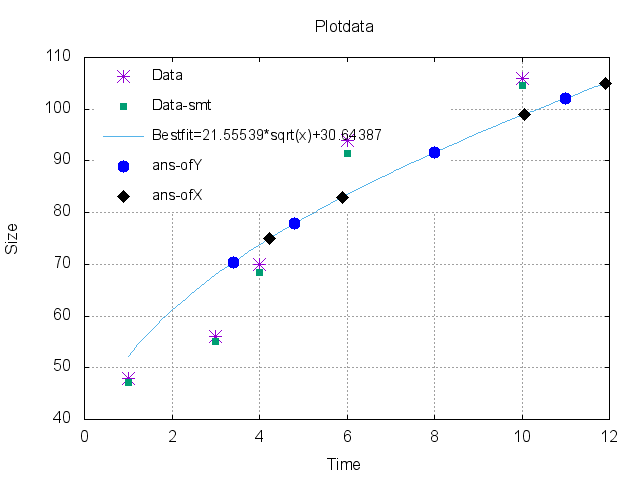Nurturing Pluto's Martians

# npm

Meet npm Pro: unlimited public & private packages + package-based permissions.Learn more »

## nsolvejs

1.1.1 • Public • Published

# Nsolvejs## Introduction

(Before JNsolve)Solve numerically equations and calculate best fit to a data array given, also provides a series of numeric routines usable.

## Features

• Nsolvejs statistical
• Nsolvejs bestfit
• Nsolvejs regulafalsi
• Nsolvejs fixedpoint
• Nsolvejs bisection
• Nsolvejs Newton-Raphson
• Nsolvejs Newton-Raphson-Higher-Order
• Nsolvejs Numerical Derivative
• Nsolvejs FindRoot

## API

### `Nsolvejs`

Initialize `Nsolvejs`

### `Numerical analysis`

#### `Nsolvejs.calculusN.D`

Object with differents numerics methods to calculate the derivative of a function.

##### `Nsolvejs.calculusN.D.NumericalDerivateof(Function,Number,Array)`

Constructor that generates the numeric derivative of `Function`=> f(x) with a `Number` => N given of divisions in an interval `Array` => [a,b].

##### `Nsolvejs.calculusN.D.NumericalDerivateof.f_x`

Instance method what is the derivative numerical of `Function` with a `Number` given of divisions in an interval `Array`.

is a aproximation to the derivative of f (df_dx) on 3 with the 1000 divisions in the interval [2,7]. Is available another method that calculate the numerical derivative calculating the dx_i in a optimazed way, dx_i=h/sqrt(1+dfdx^2) with h=(b-a)/N.

##### `Nsolvejs.calculusN.D.linear_interpolation(Array)`

Is a constructor that generates the numeric linear interpolation of data given in `Array`= [[x_1,y_2],[x_2,y_3],...[x_n,y_n]] in the interval [x_1,x_n].

##### `Nsolvejs.calculusN.D.linear_interpolation(Array).function_interpolated`

Is a instance method what is the interpolated function of `Array` given.

Is a aproximation interpolated to the `Array` = [[0,3.2],[1,4.6],[2,5.1],[4,6.9]].

#### `Nsolvejs.nsolveqn(Function, Array[,Number,Object])`

Is a method that calculate numerically the solution of `Function`=>f(x)=0 try in the interval (`Array`=>[a,b]) beginning on `Number`=>x_0 (initial point).

The `Object`is default options and are { npointsDNumeric : 1000, presicion : 0.001 , nstepsmax : 1000 , method : 'Newton_Rapshon' }. The mothods available are RegulaFalsi, bisection,fixedpoint,Newton_Raphson_Higherorder, Newton_Raphson. The rest of routines for every method are availables:

#### `Nsolvejs.calculusN.Newton_Raphson_Higherorder(Function,Array[, Number, Object])`

in every case if x_0 is undefined, is taken from a random number in interval `Array`=>[a,b]. All these methods return a object with properties Root, numSteps and method used.

#### `Nsolvejs.calculusN.findroot(Function, Array[,Number,Object])`

Is a method that calculate numerically the solution of `Function`=>f(x)=0 try in the interval (`Array`=>[a,b]) beginning on `Number`=>x_0 (initial point).

The `Object`is default options and are { npointsDNumeric : 1000, precision : 0.001 , nstepsmax : 1000 , method : 'Newton_Rapshon' }. Here, findroot try find the root of function by all methods availables in the module.

### `Data Fitting`

#### `Nsolvejs.fit.best(Array[,Array,Array,Object,Function])`Calculate the best fit using the first `Array`= [[x_1,y_1,z_1...],[x_2,y_2,z_2...],...[x_n,y_n,z_n,...]] argument as data input (if the fit is already calculated before you can pass it instead), the second `Array` = [z_1,z_2...z_m] argument are the values of x's for which is necessary calculate their y`s values respectively, the third argument are the values of "y" for which is queried the values of "x". The properties of options object are smoothing (default = True), noiseeliminate (default = True), smoothingmethod (default ='exponential' only by moment), alpha (default = 0.8) and fits_name (the fits function) to use: the availables function are inverse (a/(b+x)), linear (ax+b), exponential (a_e^(bx)), logarithmic (a+b Log(x)), polynomial (ax^2+bx+c), sqrt (a_ sqrt(x)+b) and power (ax^b), if not specified take all function availables, using (array) property specified which column of data in Array is taken to do the fist. The noiseeliminate method eliminate data that are beyond of 3.5 standard deviation from mean(99.95 % Reliability if data have a normal distribution), does that make a loop filter until that not one data is out of this limit. Return a object with the properties: ans_ofY,ans_ofX, fitUsed, fitEquationUsed, fitParamsUsed, fitPointsUsed, fitWithError and fit. The last parameter is a callback function that receive as only parameter the fit self.

## Contributing

In lieu of a formal style guide, take care to maintain the existing coding style. Add unit tests for any new or changed functionality. Lint and test your code. For any bugs report please contact to me via e-mail: jesus.edelcereceres@gmail.com.

## Licence

Permission is hereby granted, free of charge, to any person obtaining a copy of this software and associated documentation files (the "Software"), to deal in the Software without restriction, including without limitation the rights to use, copy, modify, merge, publish, distribute, sublicense, and/or sell copies of the Software, and to permit persons to whom the Software is furnished to do so, subject to the following conditions:

The above copyright notice and this permission notice shall be included in all copies or substantial portions of the Software.

THE SOFTWARE IS PROVIDED "AS IS", WITHOUT WARRANTY OF ANY KIND, EXPRESS OR IMPLIED, INCLUDING BUT NOT LIMITED TO THE WARRANTIES OF MERCHANTABILITY, FITNESS FOR A PARTICULAR PURPOSE AND NONINFRINGEMENT. IN NO EVENT SHALL THE AUTHORS OR COPYRIGHT HOLDERS BE LIABLE FOR ANY CLAIM, DAMAGES OR OTHER LIABILITY, WHETHER IN AN ACTION OF CONTRACT, TORT OR OTHERWISE, ARISING FROM, OUT OF OR IN CONNECTION WITH THE SOFTWARE OR THE USE OR OTHER DEALINGS IN THE SOFTWARE.

### Install

`npm i nsolvejs`

1,132

1.1.1

MIT

60 kB

52